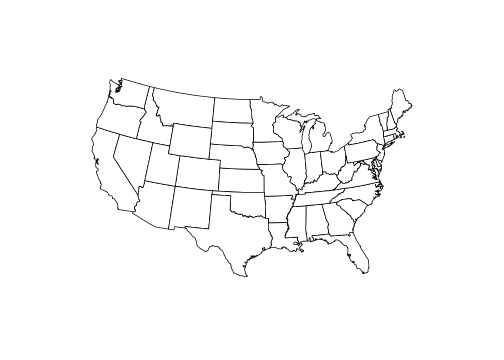# ggthemes Package

## Overview

Some extra geoms, scales, and themes for ggplot, including:

### Geoms

• geom_rangeframe : Tufte’s range frame
• geom_tufteboxplot: Tufte’s box plot

### Themes

• theme_calc: a theme based on LibreOffice Calc.
• theme_economist: a theme based on the plots in the The Economist magazine.
• theme_excel: a theme replicating the classic ugly gray charts in Excel
• theme_few: theme from Stephen Few’s “Practical Rules for Using Color in Charts”.
• theme_fivethirtyeight: a theme based on the plots at fivethirtyeight.com.
• theme_gdocs: a theme based on Google Docs.
• theme_hc: a theme based on Highcharts JS.
• theme_pander: a theme to use with the pander package.
• theme_solarized: a theme using the solarized color palette.
• theme_stata: themes based on Stata graph schemes.
• theme_tufte: a minimal ink theme based on Tufte’s The Visual Display of Quantitative Information.
• theme_wsj: a theme based on the plots in the The Economist magazine.

### Scales

• scale_colour_calc, scale_shape_calc: color and shape palettes from LibreOffice Calc.
• scale_colour_colorblind: Colorblind safe palette from http://jfly.iam.u-tokyo.ac.jp/color/.
• scale_colour_economist: colors used in plots in plots in The Economist.
• scale_colour_excel: colors from new and old Excel.
• scale_colour_few: color palettes from Stephen Few’s “Practical Rules for Using Color in Charts”.
• scale_colour_gdocs: color palette from Google Docs.
• scale_colour_hc: a theme based on Highcharts JS.
• scale_colour_solarized: Solarized colors
• scale_colour_stata, scale_shapes_stata, scale_linetype_stata: color, shape, and linetype palettes from Stata graph schemes.
• scale_colour_tableau, scale_shape_tableau: color and shape palettes from Tableau.
• scale_colour_pander, scale_fill_pander: scales to use with the pander package.
• scale_shape_cleveland, scale_shape_tremmel, scale_shape_circlefill: shape scales from classic works in visual perception: Cleveland, Tremmel (1995), and Lewandowsky and Spence (1989).
• scale_x_tufte, scale_y_tufte: x and y scales with pretty labels that also include the min and max values.

Most of these scales also have associates palettes, as used in the scales package.

### Miscellaneous

• bank_slopes: Find the optimal aspect ratio to bank slopes to 45 degrees

## Examples

library("ggplot2")
library("ggthemes")
dsamp <- diamonds[sample(nrow(diamonds), 1000), ]

### Tufte theme and geoms

Minimal theme and geoms based on plots in The Visual Display of Quantitative Information.

(ggplot(mtcars, aes(wt, mpg))
+ geom_point() + geom_rangeframe()
+ theme_tufte())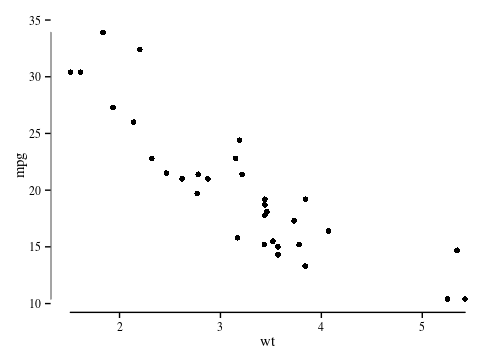The Tufte minimal boxplot.

(ggplot(mtcars, aes(factor(cyl), mpg))
+ theme_tufte(ticks=FALSE)
+ geom_tufteboxplot())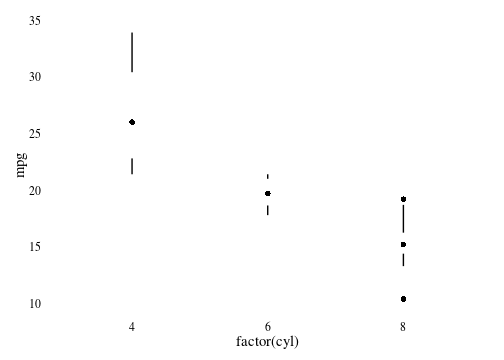### Economist theme

A theme that approximates the style of plots in The Economist magazine.

(qplot(carat, price, data=dsamp, colour=cut)
+ theme_economist()
+ scale_colour_economist()
+ ggtitle("Diamonds Are Forever"))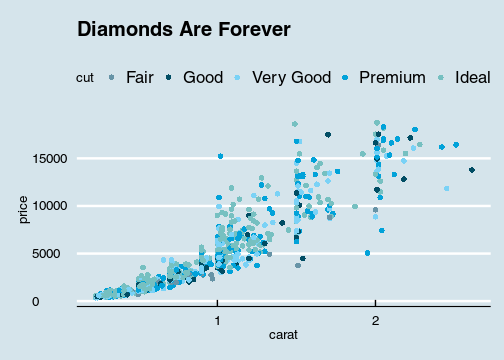### Solarized theme

A theme and color and fill scales based on the Solarized palette.

The light theme.

(qplot(carat, price, data=dsamp, colour=cut)
+ theme_solarized()
+ scale_colour_solarized("blue"))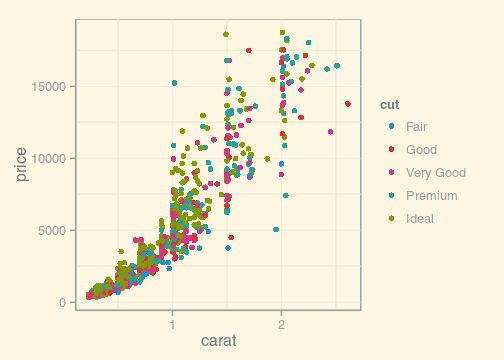The dark theme.

(qplot(carat, price, data=dsamp, colour=cut)
+ theme_solarized(light=FALSE)
+ scale_colour_solarized("red"))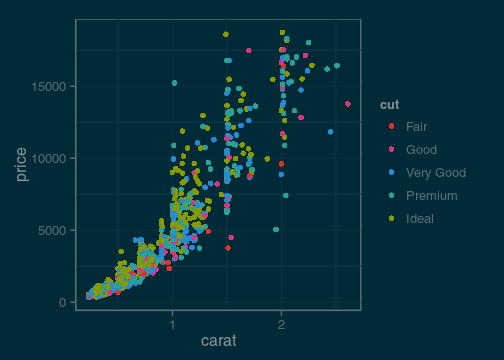An alternative theme.

(qplot(carat, price, data=dsamp, colour=cut)
+ theme_solarized_2()
+ scale_colour_solarized("blue"))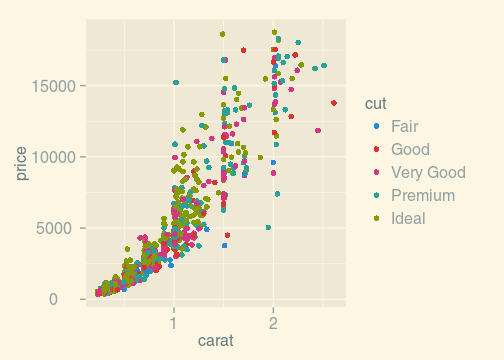### Stata theme

Themes and scales (color, fill, linetype, shapes) based on the graph schemes in Stata.

(qplot(carat, price, data=dsamp, colour=cut)
+ theme_stata()
+ scale_colour_stata()
+ ggtitle("Plot Title"))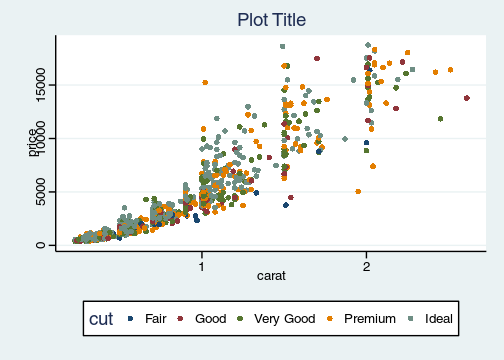### Excel 2003 theme

For that classic ugly look and feel. For ironic purposes only. 3D bars and pies not included. Please never use this theme.

(qplot(carat, price, data=dsamp, colour=cut)
+ theme_excel()
+ scale_colour_excel())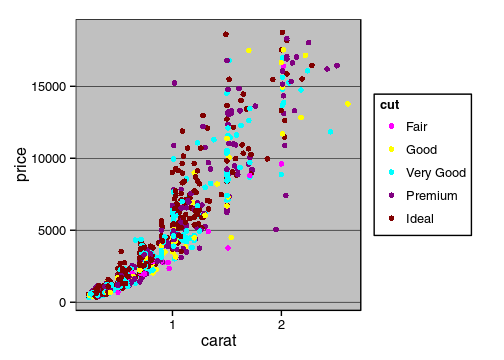(ggplot(diamonds, aes(clarity, fill=cut))
+ geom_bar()
+ scale_fill_excel()
+ theme_excel())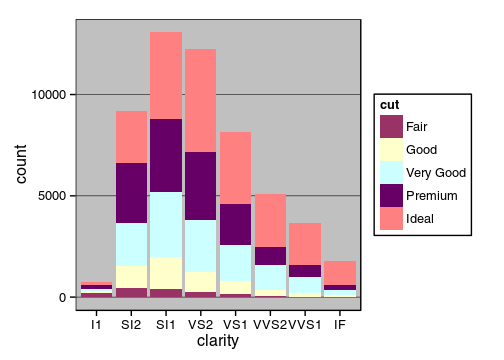### Inverse Gray Theme

Inverse of theme_gray, i.e. white plot area and gray background.

(qplot(carat, price, data=dsamp, colour=cut)
+ theme_igray())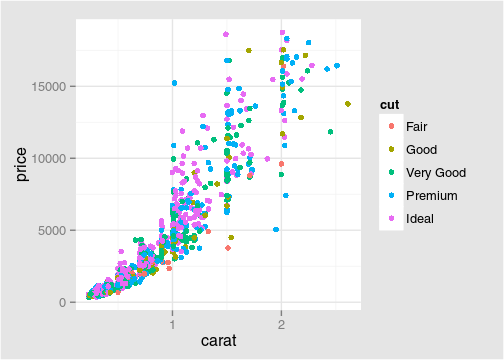### Fivethirtyeight theme

Theme and color palette based on the plots at fivethirtyeight.com.

(qplot(hp, mpg, data= subset(mtcars, cyl != 5), geom="point", color = factor(cyl))
+ geom_smooth(method = "lm", se = FALSE)
+ scale_color_fivethirtyeight()
+ theme_fivethirtyeight())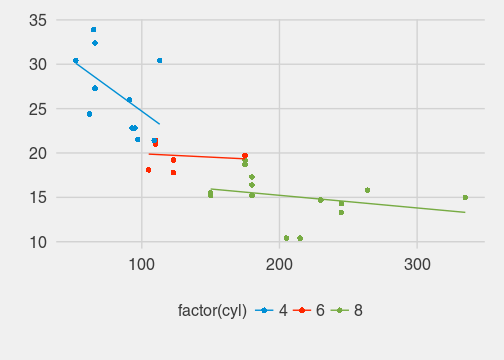### Tableau Scales

Color, fill, and shape scales based on those used in the Tableau software.

(qplot(carat, price, data=dsamp, colour=cut)
+ theme_igray()
+ scale_colour_tableau())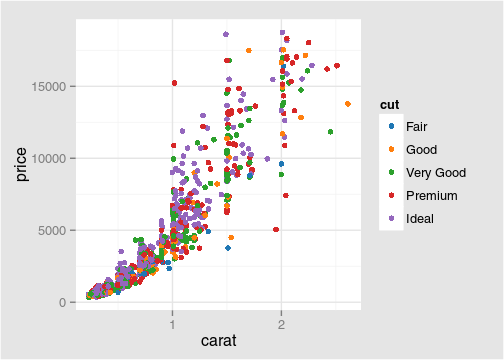(qplot(carat, price, data=dsamp, colour=cut)
+ theme_igray()
+ scale_colour_tableau("colorblind10"))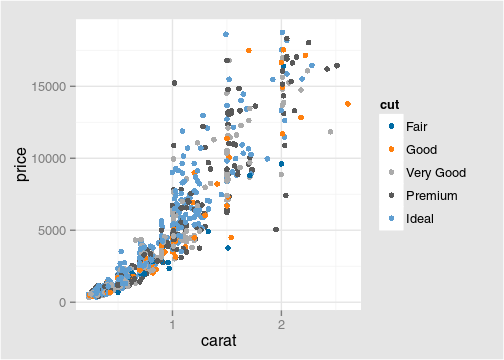### Stephen Few’s Practical Rules for Using Color …

Color palette and theme based on Stephen Few’s “Practical Rules for Using Color in Charts”.

(qplot(carat, price, data=dsamp, colour=cut)
+ theme_few()
+ scale_colour_few())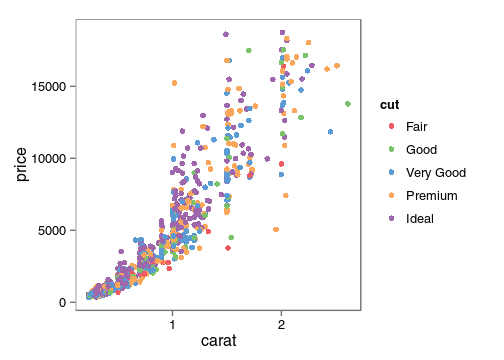### Wall Street Journal

Theme and some color palettes based on plots in the The Wall Street Journal.

(qplot(carat, price, data=dsamp, colour=cut)
+ theme_wsj()
+ scale_colour_wsj("colors6", "")
+ ggtitle("Diamond Prices"))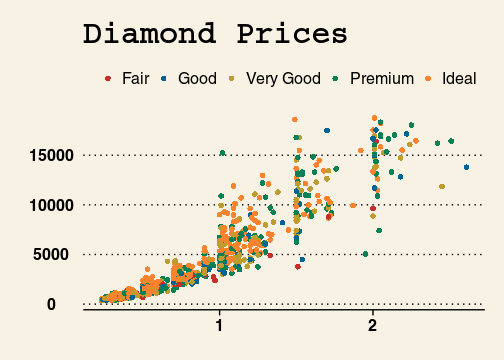### GDocs Theme

Theme and color palettes based on the defaults in Google Docs.

(qplot(carat, price, data=dsamp, colour=clarity)
+ theme_gdocs()
+ ggtitle("Diamonds")
+ scale_color_gdocs())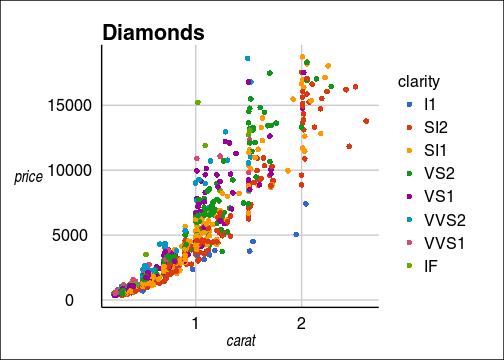### Calc Theme

Theme and color and shape palettes based on the defaults in LibreOffice Calc.

(qplot(carat, price, data=dsamp, colour=clarity)
+ theme_calc()
+ ggtitle("Diamonds")
+ scale_color_calc())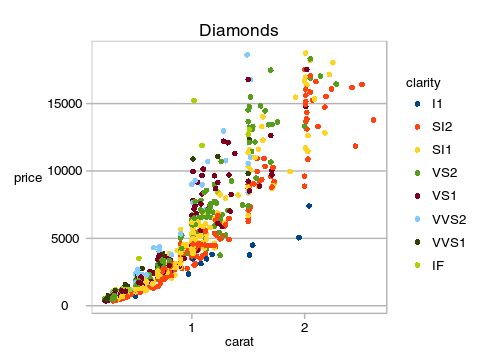### Pander Theme

Theme and color palettes based on the pander package.

(qplot(carat, price, data = dsamp, colour = clarity)
+ theme_pander()
+ scale_colour_pander())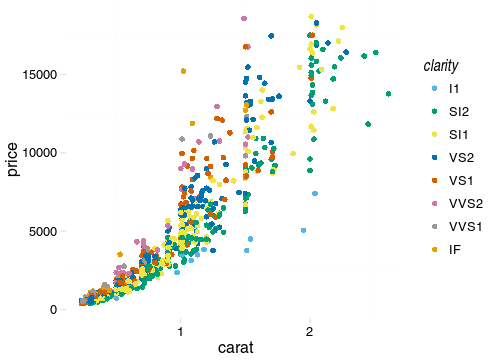(ggplot(dsamp, aes(clarity, fill = cut)) + geom_bar()
+ theme_pander()
+ scale_fill_pander())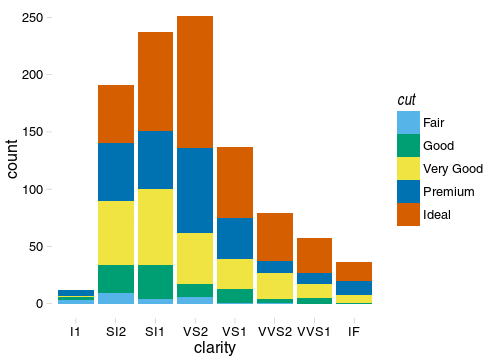### Highcharts theme

A theme that approximates the style of plots in Highcharts JS.

(qplot(carat, price, data = dsamp, colour = cut)
+ theme_hc()
+ scale_colour_hc()
+ ggtitle("Diamonds Are Forever"))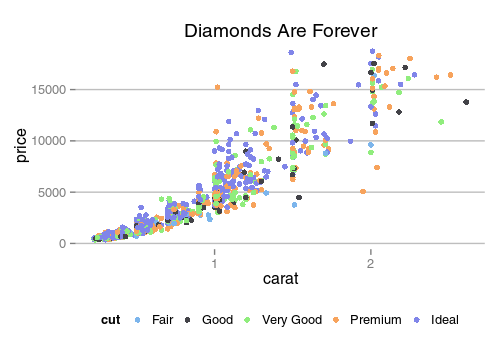(qplot(carat, price, data = dsamp, colour = cut)
+ theme_hc(bgcolor = "darkunica")
+ scale_colour_hc("darkunica")
+ ggtitle("Diamonds Are Forever"))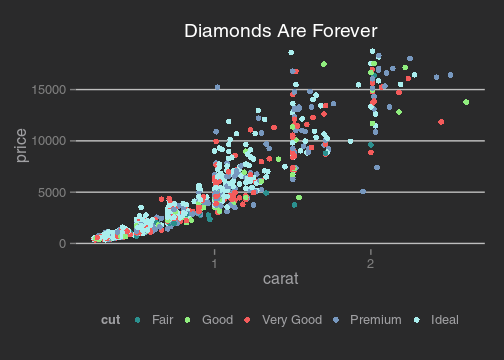dtemp <- data.frame(months = factor(rep(substr(month.name,1,3), 4), levels = substr(month.name,1,3)),
city = rep(c("Tokyo", "New York", "Berlin", "London"), each = 12),
temp = c(7.0, 6.9, 9.5, 14.5, 18.2, 21.5, 25.2, 26.5, 23.3, 18.3, 13.9, 9.6,
-0.2, 0.8, 5.7, 11.3, 17.0, 22.0, 24.8, 24.1, 20.1, 14.1, 8.6, 2.5,
-0.9, 0.6, 3.5, 8.4, 13.5, 17.0, 18.6, 17.9, 14.3, 9.0, 3.9, 1.0,
3.9, 4.2, 5.7, 8.5, 11.9, 15.2, 17.0, 16.6, 14.2, 10.3, 6.6, 4.8))
qplot(months, temp, data=dtemp, group=city, color=city, geom="line") +
geom_point(size=1.1) +
ggtitle("Monthly Average Temperature") +
theme_hc() +
scale_colour_hc()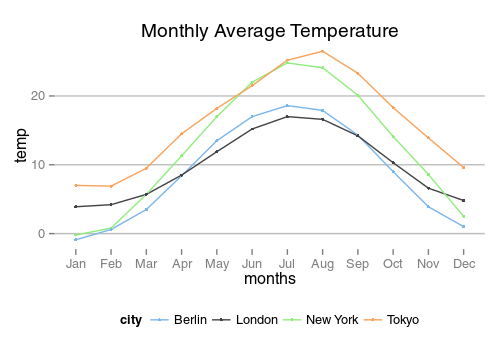qplot(months, temp, data=dtemp, group=city, color=city, geom="line") +
geom_point(size=1.1) +
ggtitle("Monthly Average Temperature") +
theme_hc(bgcolor = "darkunica") +
scale_fill_hc("darkunica")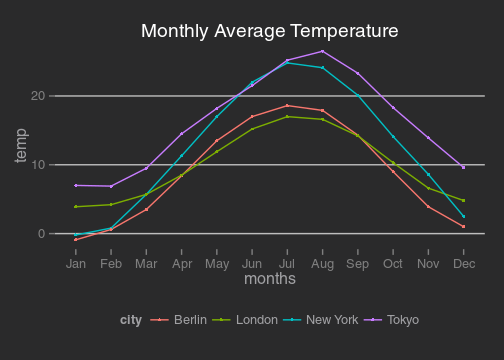## Maps theme

A theme useful for displaying maps.

library("maps")
us <- fortify(map_data("state"), region = "region")
(ggplot()
+ geom_map(data  =  us, map = us,
aes(x = long, y = lat, map_id = region, group = group),
fill = "white", color = "black", size = 0.25)
+ coord_map("albers", lat0 = 39, lat1 = 45)
+ theme_map()
)